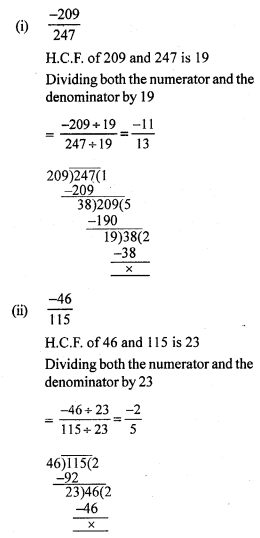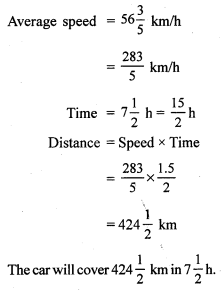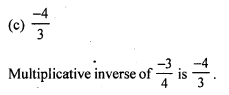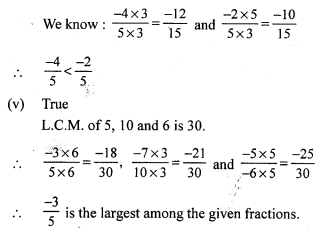# RS Aggarwal Class 7 Solutions Chapter 4 Rational Numbers CCE Test Paper

In this chapter, we provide RS Aggarwal Solutions for Class 7 Chapter 4 Rational Numbers CCE Test Paper for English medium students, Which will very helpful for every student in their exams. Students can download the latest RS Aggarwal Solutions for Class 7 Chapter 4 Rational Numbers CCE Test Paper Maths pdf, free RS Aggarwal Solutions Class 7 Chapter 4 Rational Numbers CCE Test Paper Maths book pdf download. Now you will get step by step solution to each question.

### RS Aggarwal Solutions for Class 7 Chapter 4 Rational Numbers CCE Test Paper Download PDF

Question 1.
Solution:Question 2.
Solution:Question 3.
Solution:
Let the required number be x.Question 4.
Solution:
Let the other number be x.Question 5.
Solution:Question 6.
Solution:
Let the required number be xQuestion 7.
Solution:
Length of rope = 45 mQuestion 8.
Solution:Mark (✓) against the correct answer in each of the following :
Question 9.
Solution:Question 10.
Solution:Question 11.
Solution:Question 12.
Solution:Question 13.
Solution:Question 14.
Solution:Question 15.
Solution:Question 16.
Solution:Question 17.
Solution:
(i) False.
This is because −15−11 = 1511, which lies to the right of 0.
(ii) True
(iii) True
(iv) False
L.C.M. of 5 and 3 is 15.All Chapter RS Aggarwal Solutions For Class 7 Maths

—————————————————————————–

All Subject NCERT Exemplar Problems Solutions For Class 7

All Subject NCERT Solutions For Class 7

*************************************************

I think you got complete solutions for this chapter. If You have any queries regarding this chapter, please comment on the below section our subject teacher will answer you. We tried our best to give complete solutions so you got good marks in your exam.

If these solutions have helped you, you can also share rsaggarwalsolutions.in to your friends.